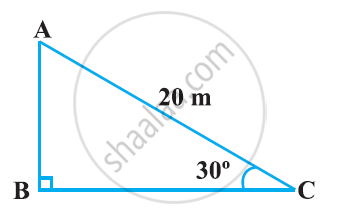Share

# A circus artist is climbing a 20 m long rope, which is tightly stretched and tied from the top of a vertical pole to the ground. Find the height of the pole, if the angle made by the rope with the ground level is 30 ° - CBSE Class 10 - Mathematics

#### Question

A circus artist is climbing a 20 m long rope, which is tightly stretched and tied from the top of a vertical pole to the ground. Find the height of the pole, if the angle made by the rope with the ground level is 30 °#### Solution

It can be observed from the figure that AB is the pole.

In ΔABC,

AB/AC  =  sin 30º

AB/20 =1/2

AB = 20/2 = 10

Therefore, the height of the pole is 10 m

Is there an error in this question or solution?

#### APPEARS IN

NCERT Solution for Mathematics Textbook for Class 10 (2019 to Current)
Chapter 9: Some Applications of Trigonometry
Ex. 9.10 | Q: 1 | Page no. 203

#### Video TutorialsVIEW ALL 

Solution A circus artist is climbing a 20 m long rope, which is tightly stretched and tied from the top of a vertical pole to the ground. Find the height of the pole, if the angle made by the rope with the ground level is 30 ° Concept: Heights and Distances.
S